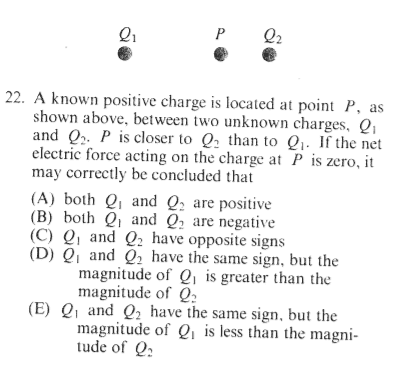# Net force acting on positive charge

ardour

## Homework StatementF= (k*q*Q)/r^2

## The Attempt at a Solution

The answer key gives the answer as D. I thought it was C. If the net electric force acting on P is zero, doesn't that mean that the force between Q1 and P and the force between Q2 and P need to cancel each other out? If you added them together, one would have to be positive, and the other would have to be negative to cancel out. Also, why should Q1 have a magnitude greater than Q2? Since Q2 is closer to P, I would have thought that it would have had a greater charge than Q1.